Home > MC2 > Chapter 11 > Lesson 11.2.4 > Problem11-71

11-71.
1. Find the probability of each event, based on the given information. Homework Help ✎

1. If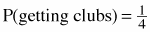, what is P(~getting clubs)?

2. If P(A) = 0.4, what is P(~A)?

3. If P(win) = x%, what is P(~win)?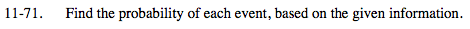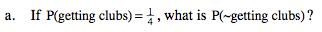These two outcomes are opposite of each other. That means that
both of these outcomes together must equal 1, or 100% probability.

$\text{What can you add to \frac{1}{4} to get 1?}$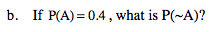See part (a).

0.6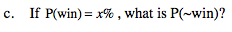If there is a 20% chance of winning, that means there is a
100% − 20% = 80% chance of not winning. Show this method
using the variable x.

(100 − x)%